/ +91 9829211106
Welcome to Vidhyarthi Darpan

# Maths 12th Previous Year Question Paper 2018 (CBSE)###### Maths 12th Previous Year Question Paper 2019 (CBSE)
November 30, 2019###### Accountancy 12th Previous Year Question Paper 2018 (CBSE)
December 3, 2019MathsSection – AQ.1. Find the value of tan-1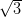-cot-1 .

Solution: We haveQ.2. If the matrix A =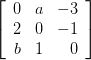is skew-symmetric, find the values of’a’ and ‘b’.

Solution:Q.3. Find the magnitude of each of the two vectors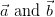, having the same magnitude such that the angle between them is 60° and their scalar product is 9/2

Solution: Let be two such vectors.Q.4. If a * b denotes the larger of ‘a’ and ‘b’ and if a o b = (a * b) + 3, then write the value of (5) o (10), where * and o are binary operations.**

Section – BQ.5. Prove that

Solution: R.H.S = sin-1(3x – 4x3)

Putting x = sin θ in R.H.S, we get

R.H.S = sin-1(3 sin θ – 4 sin3 θ)

= sin-1(sin 3θ)Q.6. Given A =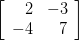compute A-1 and show that 2A-1 = 9I – A. 

Solution:Q.7. Differentiate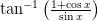with respect to x.

Solution:Q.8. The total cost C(x) associated with the pro-duction of x units of an item is given by C(x) = 0.005x3 – 0.02x2 + 30s + 5000. Find the marginal cost when 3 units are produced, where by marginal cost we mean the instantaneous rate of change of total cost at any level of output.

Solution : Cost function is given as

C(s) = 0.005x3 – 0.02x2 + 30s + 5000

Marginal cost (MC) =

= 0.005(3x2) – 0.02 (2x) + 30

= 0.015x2 – 0.04s+ 30

When x = 3,MC = 0.015(3)2 – 0.04(3) + 30

= 0.135 – 0.12 + 30

= 30.015Q.9. Evaluate,

Solution: We have,Q.10. Find the differential equation representing the family of curves y = aebx + 5, where a and b are arbitrary constants.

Solution: Given curve is

y = aebx + 5 …(i)

Differentiating (i) w.r.t. s, we getQ.11. If θ is the angle between two vectors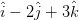and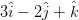, find sin θ.

Solution:Q.12. A black and a red die are rolled together. Find the conditional probability of obtaining the sum 8, given that the red die resulted in a number less than 4.

Solution : The sample space has 36 Outcomes. Let A be event that the sum of observations is 8.

∴ A = {(2, 6), (3, 5), (5, 3), (4, 4), (6, 2)}

Section – CQ.13. Using properties of determinants, prove that:

Solution:Q.14. If (x2 + y2)2 = xy, find dy/dx.

Solution: We have,

(x2 + y2)2 = xy …(i)

ORQ.14. If x = a(2θ – sin 2θ) and y = a(1 – cos 2θ), find dy/dx when θ =π ⁄3

Solution: Given, x = a (2θ – sin 2θ)  and y = a (1 – cos 2θ)

Differentiating x* and y w.r.t. θ, we getQ.15. If y = sin(sin x), prove that:

Solution: Given, y = sin (sin x)

Differentiating y w.r.t. x, we get

Differentiating again w.r.t. x, we getQ.16. Find the equations of the tangent and the normal to the curve 16x2 + 9y2 = 145 at the point (x1, y1), where x1 = 2 and y1 > 0.

Solution: Given curve is

16x2 + 9y2 = 145 ….(i)

Since (x1, y1) lies on equation (i),

OR

Q.16. Find the intervals in which the function

(a) strictly increasing,

(b) strictly decreasing.

Solution: Given function isQ.17. An open tank with a square base and vertical sides is to be constructed from a metal sheet so as to hold a given quantity of water. Show that the cost of material will be least when depth of the tank is half of its width. If the cost is to be borne by nearby settled lower income families, for whom water will be provided, what kind of value is hidden in this question ?

Solution: Let the length, breadth and height of the open tank be x, x and y units respectively.

Then, Volume (V) = x2y …(i)

Area is minimum, thus cost is minimum when x = 2y.

i.e., depth of tank is half of the width.

Value : Any relevant value.Q.18. Find

Solution:Q.19. Find the particular solution of the differential equation ex tan ydx + (2 – ex) sec2 ydy = 0, given that y =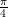when x = 0.

Solution: Given differential equation is,

ORQ.19. Find the particular solution of the differential equation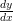+ 2y tan x = sin x, given than y = 0 when x =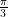.

Solution: Given differential equation is,

Hence, particular solution is

y sec2 x = sec x – 2

or y = cos x – 2 cos2xQ.20. Let Find a vector d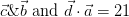which is perpendicular to both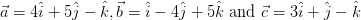.

Solution: GivenQ.21. Find the shortest distance between the linesand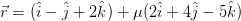.

Solution: Gives lines are,Q.22. Suppose a girl throws a die. If she gets 1 or 2, she tosses a coin three times and notes the number of tails. If she gets 3, 4, 5 or 6, she tosses a coin once and notes whether a ‘head’ or ‘tail’, is obtained. If she obtained exactly one “tail7, what is the probability that she threw 3, 4, 5 or 6 with the dice?

Solution : Let E1 be the event that girl gets 1 or 2 on the roll and E2 be the event that girl gets 3, 4, 5, or 6 on the roll of a die.

Let A be the event that she gets exactly one tail. If she tossed coin 3 times and gets exactly one tail then possible outcomes are HTH, HHT, THH.

= 8/11Q.23. Two numbers are selected at random (without replacement) from the first five positive integers. Let X denote the larger of the two numbers obtained. Find the mean and variance of X.

Solution: First five positive integers are 1, 2, 3, 4, 5. We select two positive numbers in 5 × 4 = 20 ways. Out of these, two numbers are selected at random. Let X denote larger of the two selected numbers. Then, X can have values 2, 3, 4 or 5.

P(X = 2) – P (larger no. is 2) = {(1, 2) and (2, 1)}

Therefore, mean and variance are 4 and 1 respectively.Q.24. Let A = {x ϵ Z : 0 ≤ x ≤ 12}. Show that R = {(a, b): a, b ϵ A, | a – b | is divisible by 4} is an equivalence relation. Find the set of all elements related to 1. Also write the equivalence class .

Solution: Given, R = {(a, b): a, b ϵ A, | a – b | is divisible by 4}

Reflexivity: For any a ϵ A

| a – a | = 0, which is divisible by 4

(a, a) ϵ R

So, R is reflexive.

Symmetry: Let (a, b) ϵ R

⇒ | a – b | is divisible by 4

⇒ | b – a | is divisible by 4 [ ∵ | a – b | = | b – a | ]

⇒ (b, a) ϵ R

So, R is symmetric.

Transitive : Let (a, b) ϵ R and (b, c) ϵ R

⇒ | a – b | is divisible by 4<br> ⇒ | a – b | = 4k

∴ a – b = ± 4k, k ϵ Z …(i)

Also, |b – c| is divisible by 4

⇒ |b – c| = 4m

∴ b – c = ±4m, m ϵ Z …(ii)

a – b + b – c = ±4 (k + m)

⇒ a – c = ±4 (k + m)

|a – c | is divisible by 4,

⇒ (a, c) ϵ R

So, R is symmetric.

⇒ R is reflexive, symmetric and transitive.

∴ R is an equivalence relation.

Let x be an element of R such that (x, 1) ϵ R

Then | x – 1| is divisible by 4

x – 1 = 0, 4, 8, 12,

⇒ -x = 1, 5, 9 (∵ x ≤ 12)

∴ Set of all elements of A which are related to 1 are {1, 5, 9}.

Equivalence class of 2 i.e.

 = {(a, 2): a ϵ A, |a – 2| is divisible by 4}

⇒ | a – 2| = 4k(k is whole number, k ≤ 3)

⇒ a = 2, 6, 10

Therefore, equivalence class  is {2, 6, 10]

ORQ.24. Show that the function f : R → R defined by f(x) =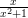, ∀x ϵ R is neither one-one nor onto. Also,if g: R → R is defined as g(x) = 2x – 1, find fog(x).

Solution:Q.25. If A = , find A-1. Use it to solve the system of equation:

2x – 3y + 5z = 11

3x + 2y – 4z = -5

x + y – 2z = -3

Solution:

ORQ.25. Using elementary row transformations, find the inverse of the matrix:

We know that, A = IA

Q.26. Using integration, find the area of the region in the first quadrant enclosed by the X-axis, the line y = x and the circle x2 + y2 = 32.

Solution: Given curve isQ.27. Evalute

Solution:

ORQ.27. Evaluate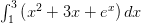as the limit of the sum.

Solution:Q.28. Find the distance of the point (-1, -5, -10) from the point of intersection of the line

Solution:  Equation of line isQ.29. A factory manufactures two types of screws A and B, each type requiring the use of two machines, an automatic and a hand-operated. It takes 4 minutes on the automatic and 6 minutes on the hand-operated machines to manufacture a packet of screws ‘A’ while it takes 6 minutes on the automatic and 3 minutes on the hand- operated machine to manufacture a packet of screws ‘B’. Each machine is available for at most 4 hours on any day. The manufacturer can sell a packet of screws ‘A’ at a profit of 70 paise and screws ‘B’ at a profit of ₹1. Assuming that he can sell all the screws he manufactures, how many packets of each type should the factory owner produce in a day in order to maximize his profit ? Formulate the above LPP and solve it graphically and find the maximum profit

Solution: Let the number of packets of screw ‘A’ manufactured in a day be x and that of screw B be y.

Plotting the points on the graph, we get the feasible region OABC as shown (Shaded).

Hence, profit will be maximum if company produces 30 packets of screw A and 20 packets of screw B and maximum profit = ₹ 41.